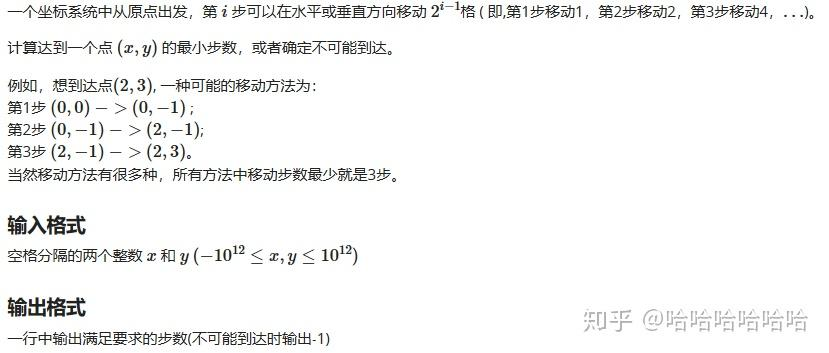# 2019级计科第一次实训Problem E

10175101159 edited 10 月前

### Problem E 计算步数从平面直角坐标系的点 $(0,0)$ 出发移动有限步，第 $i$ 步可以沿 $x$ 轴或 $y$ 轴正向或反向移动 $2^{i-1}$ 个单位长度。给定点 $(x_0,y_0)(-10^{12}\le x_0,y_0\le10^{12})$ ，问是否存在某种移动方法的终点恰为 $(x_0,y_0)$ ，如果存在，输出所有可行方法中的最少步数，否则输出 $-1$ 。

例如，输入 $(3,2)$ ，因为最少移动三步（ $(0,0)\rightarrow(-1,0)\rightarrow(-1,2)\rightarrow(3,2)$ ）到达，所以输出 $3$ ；再如输入 $(3,0)$ ，因为最少移动两步（ $(0,0)\rightarrow(1,0)\rightarrow(3,0)$ ）到达，所以输出 $2$ ；又如输入 $(-1,-1)$ ，因为不存在可行的方法到达，所以输出 $-1$ 。

观察题给样例，不难猜测可行与否与输入的奇偶性有关，有如下证明：考虑横纵坐标和的奇偶性，初始位于 $(0,0)$ ，横纵坐标和 $0+0=0$ 为偶，之后除了第一步移动 $1$ 会改变其奇偶性，此后移动 $2,4,8,16,\cdots$ 都不改变奇偶性。因此除非输入 $(0,0)$ ，只要输入的横纵坐标和为偶数都无解。此外，输入的正负是没有区别的，对水平或垂直方向全部取反向的走法就能使结果变为相反数。故下面只研究输入非负且一奇一偶的情形。

联想到 $A$ 题的平衡三进制表示，沿 $x,y$ 坐标轴正向走记 $+$ ，负向记 $-$ ，把走法表示为两个平衡二进制数，分别表示水平和垂直方向。为方便起见，我们把数位反过来从低位到高位写（从左到右每一位的权重分别是 $1,2,4,8,\cdots$ ，每个数位可以是 $-1,0,1$ ）。举个例子，前述 $(0,0)\rightarrow(3,2)$ 的走法被表示为下面两个平衡二进制数：$x=-1\times1+0\times2+1\times4,y=0\times1+1\times2+0\times4$

不难理解，这两个平衡二进制数 $x,y$ 转为十进制后恰好就是输入的 $x_0,y_0$ 。而且因为每一步要么水平移动，要么垂直移动，所以对于每个数位的数码，这两个平衡二进制数都是一个 $0$ ，另一个 $\pm1$ （ $\otimes$ ）。（这两个结论侧面反映了输入的 $x_0,y_0$ 是一奇一偶的才有解）

根据结论（ $\otimes$ ）， $x,y$ 最低位的数码一个 $0$ ，另一个 $\pm1$ ，不妨假设 $x$ 最低位是 $0$ ，那么 $y$ 最低位就是 $\pm1$ 。现在把最低位置零，$x$ 仍是 $x$ ， $y$ 变成了 $y+1$（最低位是 $-1$ ）或 $y-1$（最低位是 $1$ ），这样 $x,y$ 都是偶数了且只有权重为 $2,4,8,\cdots$ 的数位有效。权重为 $2,4,8,\cdots$ 的数位怎么同原来权重为 $1,2,4,8,\cdots$ 的数位产生联系呢？只要把每个数位的权重都减半就好了，也就相当于把偶数 $x,y\pm1$ 变为 $\frac{x}{2},\frac{y\pm1}{2}$ 。再回到结论（ $\otimes$ ），现在新的 $\frac{x}{2},\frac{y\pm1}{2}$ 也是每一位一个 $0$ ，另一个 $\pm1$ ，权重也回到了 $1,2,4,\cdots$ ，所以还是一奇一偶的。注意到 $\frac{y+1}{2}$ 和 $\frac{y-1}{2}$ 恰好相差 $1$ ，它们是一奇一偶的，而且 $\frac{x}{2}$ 的奇偶性是随输入确定的。

这样就柳暗花明了，根据 $\frac{x}{2}$ 的奇偶性我们可以确定前面是$\frac{y+1}{2}$ 还是 $\frac{y-1}{2}$ ，也意味着我们能够确定先前把最低位置零时 $y$ 是变成了 $y+1$ 还是 $y-1$ ，即我们能够确定 $y$ 的最低位是 $-1$ 还是 $1$ 。

上面的论证说明了什么？如果我们假设输入的 $x,y$ 是一奇一偶且有解的，我们就能用奇偶性确定两个平衡二进制数的最低位。而因为去掉最低位（变成$\frac{x}{2},\frac{y\pm1}{2}$）后我们又回到了与初始完全类似的情境，所以我们可能利用循环逐步确定两个平衡二进制数的每一位。“能够从合法输入确定平衡二进制数的每一位”这个事实还暗含了“如果解存在，那么解唯一”这个结论。所以我们只要进行这个循环，如果过程中出现矛盾则说明原输入无解，否则我们能得到唯一解（甚至还能得到解的每一步具体走法），也就是题目要求的最优解。

#include<bits/stdc++.h>
using namespace std;
typedef long long ll;

ll x,y;

int main()
{
cin>>x>>y;
if(x < 0)x = -x;
if(y < 0)y = -y;

int ans = 0;
while(1){
if(x==0 && y==0)break;
++ans;
if(abs(x+y) == 1)break;
if(x%2 == y%2){ // 同奇偶则不行
cout<<-1;
return 0;
}
if(x % 2){ // 交换，不妨假设x偶y奇
x ^= y,y ^= x,x ^= y;
}
x /= 2; // x最低位是0,y最低位是1或-1

// 但两种可能中，x,y抹掉最低位得到的偶数再/2还得是1奇1偶
ll t1 = (y-1)/2,t2 = (y+1)/2;
if(x%2 != t1%2)y = t1;
else y = t2;
}
cout<<ans;
return 0;
}